Session Prep: Download the dataset we will be working with for this session: Session_1.txt
This dataset contains experimental data on the pharmacokinetics of theophylline, a drug used in the treatment of COPD and asthma. Here are the descriptions of each of the variables:

Subject - a number identifying the subject on whom the observation was made. The ordering is by increasing maximum concentration of theophylline observed.
Wt - weight of the subject (kg).
Dose - dose of theophylline administered orally to the subject (mg/kg).
Time - time since drug administration when the sample was drawn (hr).
conc - theophylline concentration in the serum sample (mg/L).

# Part 1: Reading and previewing data from a text file

Just like last time, let’s read in our dataset, “Session_1.txt”. Last session, we learned two ways to import data:
This
That

1. You can specify a path for your file:
``Session_1 <- read.delim("~/Desktop/Session_1.txt")``
1. Or choose the file using a browser window:
``Session_1 <- read.delim(file.choose())``

Now let’s preview our data and move on to practicing R logical operators! You can do this by using the `View()` function. If you’re feeling really fancy, try using the tab-autocomplete feature to enter the file name:

``View(Session_1) ``
1. Help! If you need help with any function you can use the `help()` function or the `?` notation:
``````help(View)
?View``````

# Part 2: Using logical operators in R

Here are the logical operators we will be using today:

operator meaning
< less than
<= less than or equal to
> greater than
>= greater than or equal to
== exactly equal to
!= not equal to

Now that we have the dataset uploaded, let’s give some of these operators a spin. But first, we need to figure out how to access some of our data. From last time, we learned how to list a particular column in a dataset. For example, if I wanted the “weight” (Wt) column of the Session_1 dataset, I would use the following command:

``Session_1\$Wt``

And the output would look something like this:

``````   79.6 79.6 79.6 79.6 79.6 79.6 79.6 79.6 79.6 79.6 79.6
 72.4 72.4 72.4 72.4 72.4 72.4 72.4 72.4 72.4 72.4 72.4
 70.5 70.5 70.5 70.5 70.5 70.5 70.5 70.5 70.5 70.5 70.5
 72.7 72.7 72.7 72.7 72.7 72.7 72.7 72.7 72.7 72.7 72.7
 54.6 54.6 54.6 54.6 54.6 54.6 54.6 54.6 54.6 54.6 54.6
 80.0 80.0 80.0 80.0 80.0 80.0 80.0 80.0 80.0 80.0 80.0
 64.6 64.6 64.6 64.6 64.6 64.6 64.6 64.6 64.6 64.6 64.6
 70.5 70.5 70.5 70.5 70.5 70.5 70.5 70.5 70.5 70.5 70.5
 86.4 86.4 86.4 86.4 86.4 86.4 86.4 86.4 86.4 86.4 86.4
 58.2 58.2 58.2 58.2 58.2 58.2 58.2 58.2 58.2 58.2 58.2
 65.0 65.0 65.0 65.0 65.0 65.0 65.0 65.0 65.0 65.0 65.0
 60.5 60.5 60.5 60.5 60.5 60.5 60.5 60.5 60.5 60.5 60.5``````

But what about getting access to a particular value? It’s easy! Just provide the row and column coordinates, and out pops your value. For example, if I wanted to get the value in row 3, column 4 of the Session_1 dataset, I would enter:

``Session_1[3,4] ``

And the output should look like this:

`` 0.57``

Let’s break that last bit down for you. The bracket notation allows you to select specific rows and columns from a data frame. The basic syntax is data.frame[rows,columns]. If you don’t specify either rows or columns you’ll get all rows or columns. Try these commands, what do you get?

``````Session_1[3,]
Session_1[,2]``````

You can also specify ranges of numbers using a colon. For example, if I wanted the values in rows 3-6 in column 4, I would write:

``Session_1[3:6,4]``

And the output should look like this:

`` 0.57 1.12 2.02 3.82``

There are many other ways to select parts of your data, but that will do for now. Now let’s do some simple R logic!

Let’s start by checking if the value in row 1, column 1 is equal to row 2, column 1. To do this, we would enter the following:

``Session_1[1,1] == Session_1[2,1]  ``

And we should get the following output:

`` TRUE``

Turns out that the data in rows 1 and 2 both belong to Subject 1! Good thing we checked :-) legend(“topright”, legend = c(“SCARLET FEVER”, “DIPHTHERIA”),

IMPORTANT: make sure you use both the equal signs (`==`) when checking if two values are equal. If you use a single equal sign, you will re-assign the value on the right side of the =’s to the left side’s position in the dataset!
Now let’s try checking if two values are not equal. Let’s start by checking if the value in row 1, column 1 is equal to row 12, column 1. To do this, we would enter the following:

``Session_1[1,1] != Session_1[12,1]``

And we should get the following output:

`` TRUE``

Indeed, the data in row 12 belongs to subject 2. So when we compared the value in Session_1[1,1] (which was “1”), with value in Session_1[12,1] (which was “2”), it is true that these values are not equal.
So now let’s do something a little more practical with the remaining logical operators. I have a question for you: Over the course of this experiment, which subject had a higher maximum drug concentration in their bloodstream, subject 1 or subject 2? To answer this question, let’s incorporate two concepts you’ve already learned about, `max()` and selecting a range of data. We’ll also use the logical operator `>=` to check which one is higher. Believe it or not, we can do all of this in one line! See for yourself:

``max(Session_1[1:11,5]) >= max(Session_1[12:22,5])``

And we should get the following output:

`` TRUE``

Basically all we’re telling R to do is the following:
1. Find the maximum value in row 1 thru 11 in column 5 (which is the concentration data for subject 1)
2. Find the maximum value in row 12 thru 22 in column 5 (which is the concentration data for subject 2)
3. Compare the two maximum values to see if subject 1’s is higher than subject 2’s. Now imagine going through thousands of rows of data by hand looking for maximum values to compare! Phew! But we’re not done yet! Let’s just say (for fun) that the toxic concentration of theophylline in the bloodstream is 10.00 mg/kg. Does subject 1’s theophylline levels ever go past that value? Let’s check if he/she is safe (if subject 1’s maximum concentration is less than or equal to the toxic threshold of 10.00 mg/kg):

``max(Session_1[1:11,5]) <= 10.00``

And we should get the following output:

`` FALSE``

Uh oh, looks like we’ve passed the threshold! Perhaps we should alert the researchers to monitor subject 1 for theophylline overdose symptoms (cardiotoxicity and neurotoxicity). Finally, instead of specifying column numbers and row numbers (e.g. Session_1[2,5]), we can use logical criteria and column names. Using the above example we can select the subjects with doses greater than the threshold:

``````Session_1[Session_1\$conc >= 10, c("Subject","conc")]
Session_1[Session_1\$conc >= 10, c(1,5)]``````

Notice that we used the threshold to select the rows (Session_1\$conc >= 10) and we selected two columns (c(“Subject”,“conc”)). You may be wondering about the syntax for selecting multiple columns. You can specify either a list of column names or a list of column numbers. Additionally, to make your code more readable and reusable, you can assign the row and column selection criteria to objects:

``````whichRows <- Session_1\$conc >= 10
whichCols <- c("Subject","conc")
Session_1[whichRows,whichCols]``````

## Summary Quiz 1

Use what you’ve learned about logical operators and subsetting data frames with the bracket notation (answers at end).
1. Is the dose for subject 1 greater than, less than, or equal to the dose for subject 2? 2. Dose subject 4 weigh more than subject 6?
3. Is the mean concentration for subject 5 greater than, less than, or equal to the mean concentration for subject 7?
4. Is 4 not equal to 5 (prove it to yourself)?
5. What is the average concentration for Subject 7?
6. What is the minimum concentration when the time is greater than 5 minutes?
7. Which subject corresponds to the minimum concentration found in #6?

# Part 2: Sorting and ordering data

In this section we will introduce 2 functions: `sort()` and `order()`. Briefly, this is what each function does:
`sort()`: returns back a sorted list of values from a data range
`order()`: returns back a sorted list of row numbers from a data range (the row numbers that the sorted values came from)
This distinction is very important as we will soon see.
Let’s say we wanted a sorted list of the dosages given to the subjects. We would simply sort the specified column in our data set:

``sort(Session_1\$Dose)``

And we should get the following output:

``````   3.10 3.10 3.10 3.10 3.10 3.10 3.10 3.10 3.10 3.10 3.10
 4.00 4.00 4.00 4.00 4.00 4.00 4.00 4.00 4.00 4.00 4.00
 4.02 4.02 4.02 4.02 4.02 4.02 4.02 4.02 4.02 4.02 4.02
 4.40 4.40 4.40 4.40 4.40 4.40 4.40 4.40 4.40 4.40 4.40
 4.40 4.40 4.40 4.40 4.40 4.40 4.40 4.40 4.40 4.40 4.40
 4.53 4.53 4.53 4.53 4.53 4.53 4.53 4.53 4.53 4.53 4.53
 4.53 4.53 4.53 4.53 4.53 4.53 4.53 4.53 4.53 4.53 4.53
 4.92 4.92 4.92 4.92 4.92 4.92 4.92 4.92 4.92 4.92 4.92
 4.95 4.95 4.95 4.95 4.95 4.95 4.95 4.95 4.95 4.95 4.95
 5.30 5.30 5.30 5.30 5.30 5.30 5.30 5.30 5.30 5.30 5.30
 5.50 5.50 5.50 5.50 5.50 5.50 5.50 5.50 5.50 5.50 5.50
 5.86 5.86 5.86 5.86 5.86 5.86 5.86 5.86 5.86 5.86 5.86``````

Notice that these are the values that were contained in each row.
By the way, we can also sort the dose by decreasing value. We just need to set the decreasing argument to `TRUE`. Where was the decreasing argument in example 1? Well, if we don’t explicitly define it as `TRUE`, it will assume it to be `FALSE`. Therefore, the default state for the `sort()` function is ascending order. Let’s try it out:

``sort(Session_1\$Dose, decreasing = TRUE)``

And we should get the following output:

``````   5.86 5.86 5.86 5.86 5.86 5.86 5.86 5.86 5.86 5.86 5.86
 5.50 5.50 5.50 5.50 5.50 5.50 5.50 5.50 5.50 5.50 5.50
 5.30 5.30 5.30 5.30 5.30 5.30 5.30 5.30 5.30 5.30 5.30
 4.95 4.95 4.95 4.95 4.95 4.95 4.95 4.95 4.95 4.95 4.95
 4.92 4.92 4.92 4.92 4.92 4.92 4.92 4.92 4.92 4.92 4.92
 4.53 4.53 4.53 4.53 4.53 4.53 4.53 4.53 4.53 4.53 4.53
 4.53 4.53 4.53 4.53 4.53 4.53 4.53 4.53 4.53 4.53 4.53
 4.40 4.40 4.40 4.40 4.40 4.40 4.40 4.40 4.40 4.40 4.40
 4.40 4.40 4.40 4.40 4.40 4.40 4.40 4.40 4.40 4.40 4.40
 4.02 4.02 4.02 4.02 4.02 4.02 4.02 4.02 4.02 4.02 4.02
 4.00 4.00 4.00 4.00 4.00 4.00 4.00 4.00 4.00 4.00 4.00
 3.10 3.10 3.10 3.10 3.10 3.10 3.10 3.10 3.10 3.10 3.10``````

Now let’s say we wanted to sort a data frame (Session_1) according to a sorted list of values. Well, R doesn’t know how to do this right off the bat. For instance, in our output above, the first 11 values are all the same. Which row is which?? Fear not! We can simply tell R to sort the whole data frame by a particular order of rows that corresponds to our sorted list. But we’ll have to use a new function, `order()`. Let’s try it out using dose again.

``order(Session_1\$Dose)``

And we should get the following output:

``````    89  90  91  92  93  94  95  96  97  98  99  56  57
  58  59  60  61  62  63  64  65  66   1   2   3   4
   5   6   7   8   9  10  11  12  13  14  15  16  17
  18  19  20  21  22  34  35  36  37  38  39  40  41
  42  43  44  23  24  25  26  27  28  29  30  31  32
  33  78  79  80  81  82  83  84  85  86  87  88 111
 112 113 114 115 116 117 118 119 120 121  67  68  69
  70  71  72  73  74  75  76  77 122 123 124 125 126
 127 128 129 130 131 132 100 101 102 103 104 105 106
 107 108 109 110  45  46  47  48  49  50  51  52  53
  54  55``````

Notice that the output now is the row numbers that correspond to our sorted values for dose. Rows 89-99 correspond to value 3.10, just like in our `sort()` example.
Now let’s see it in action! We will be sorting the Session_1 data frame by the dose order (don’t forget the “,” at the end).

``Session_1[order(Session_1\$Dose),]``

And we should get the following output:

``Session_1[order(Session_1\$Dose),]``

Check it out! Rows 89-99 are at the top of the list, just like promised :-) But what the heck was that comma? How did R know to apply that list of ordered rows to all my columns? Well, R is smart like that. If you simply leave the comma, with no specified columns, it will assume you are referencing all the columns in the data frame.

## Summary Quiz 2

Here are a few more questions to practice sorting data
1. Sort the `Session_1` data frame by the Time variable and store it in a new object called sorted.
2. Sort the `Session_1` data frame by the Dose and conc variables and store it in a new object called sorted2.
3. Sort the `Session_1` data frame from the largest to smallest values and store it in a new object called sorted3.

# Part 3: Scatter plots, Line graphs, Box plots, Bar charts, and Histograms

Last session we learned how to produce a simple scatter plot with our data. The function `plot()` contained the x and y coordinates for arguments. For our purposes today, let’s plot concentration vs. time data for subject 1 (rows 1-11) :

``plot(Session_1\$Time[1:11],Session_1\$conc[1:11])``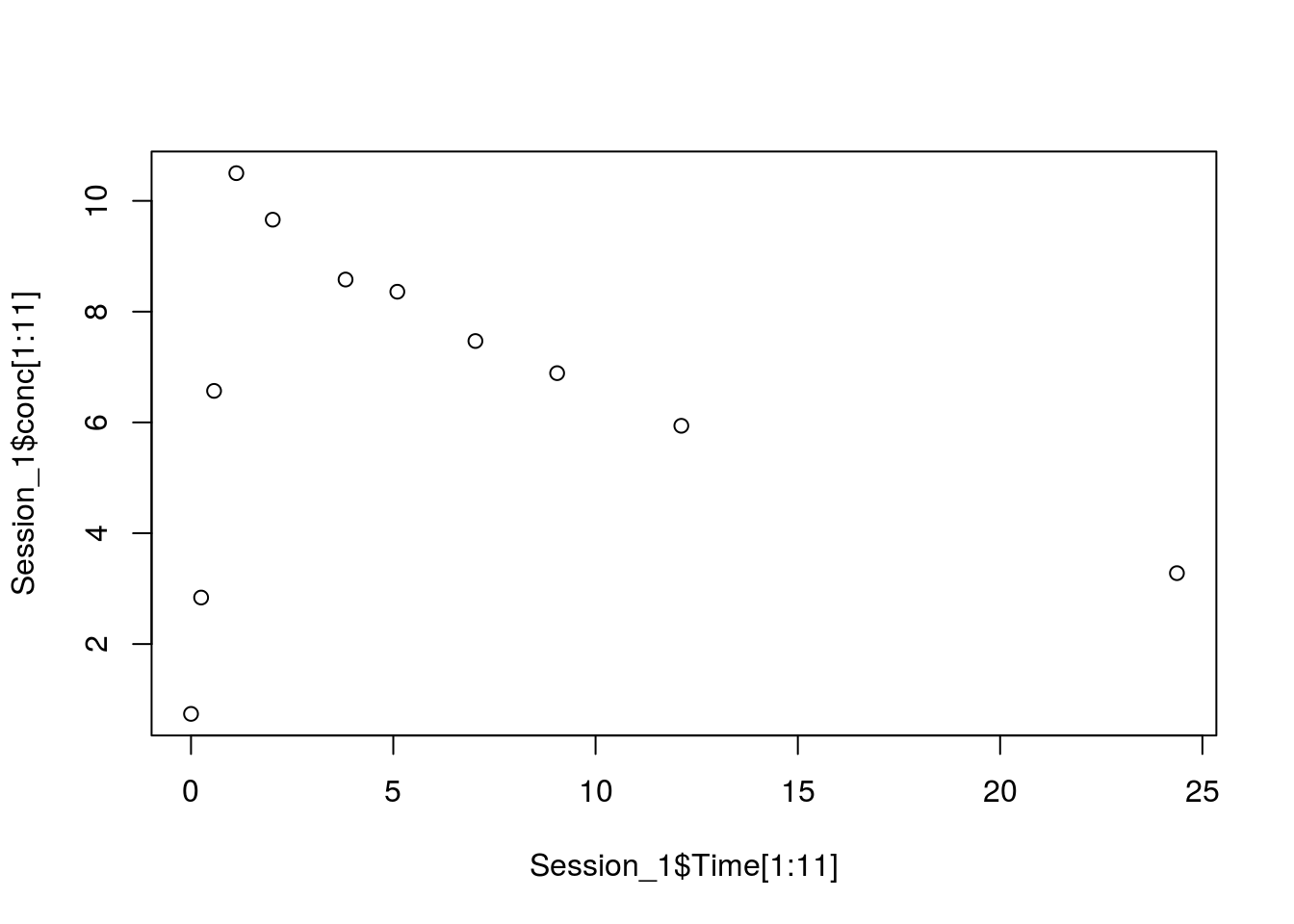Notice how we don’t have to specify the column in the brackets. That’s because we’re already in the column we want (it’s specified by the ‘Time’ or ‘conc’ after the ‘\$’ sign).
To make a line plot, we can still use the `plot()` function, we just need to specify that it will be a line graph. Notice that we added the ‘type’ argument in our function. Here, “l” stands for line. There are other options for other types of graphs too. Let’s try it out:

``plot(Session_1\$Time[1:11],Session_1\$conc[1:11], type="l")``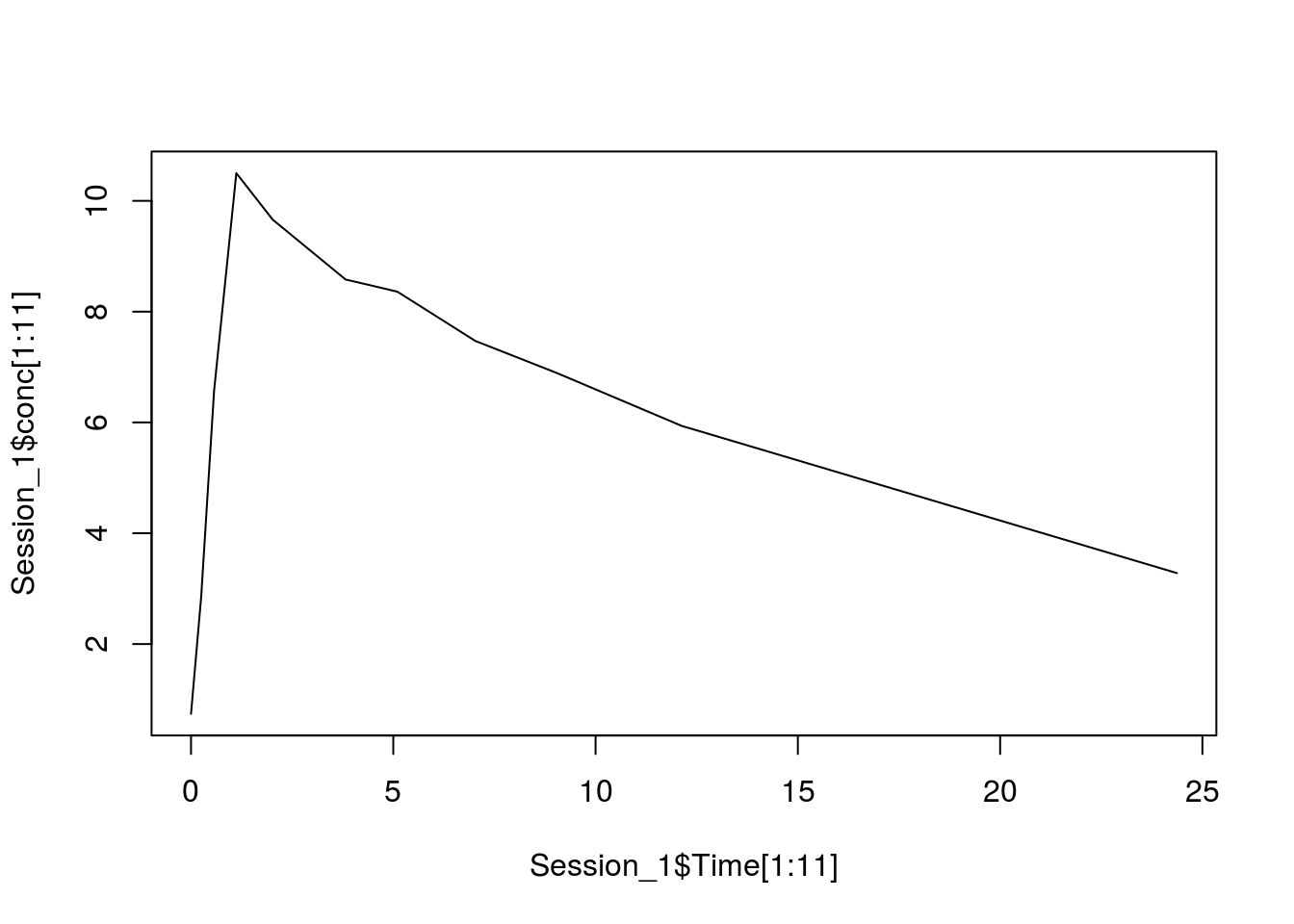That looks like a pretty good graph so far, but let’s do one better. This time, let’s add in a nice title, as well as some axes labels. We can do this by specifying more arguments, this time using: main, xlab, ylab, which specify the title, x-axis, and y-axis, respectively. The code should look something like this:

``plot(Session_1\$Time[1:11],Session_1\$conc[1:11], type="l", main="Concentration over Time", xlab="Time (hours)", ylab="Concentration (mg/kg)")``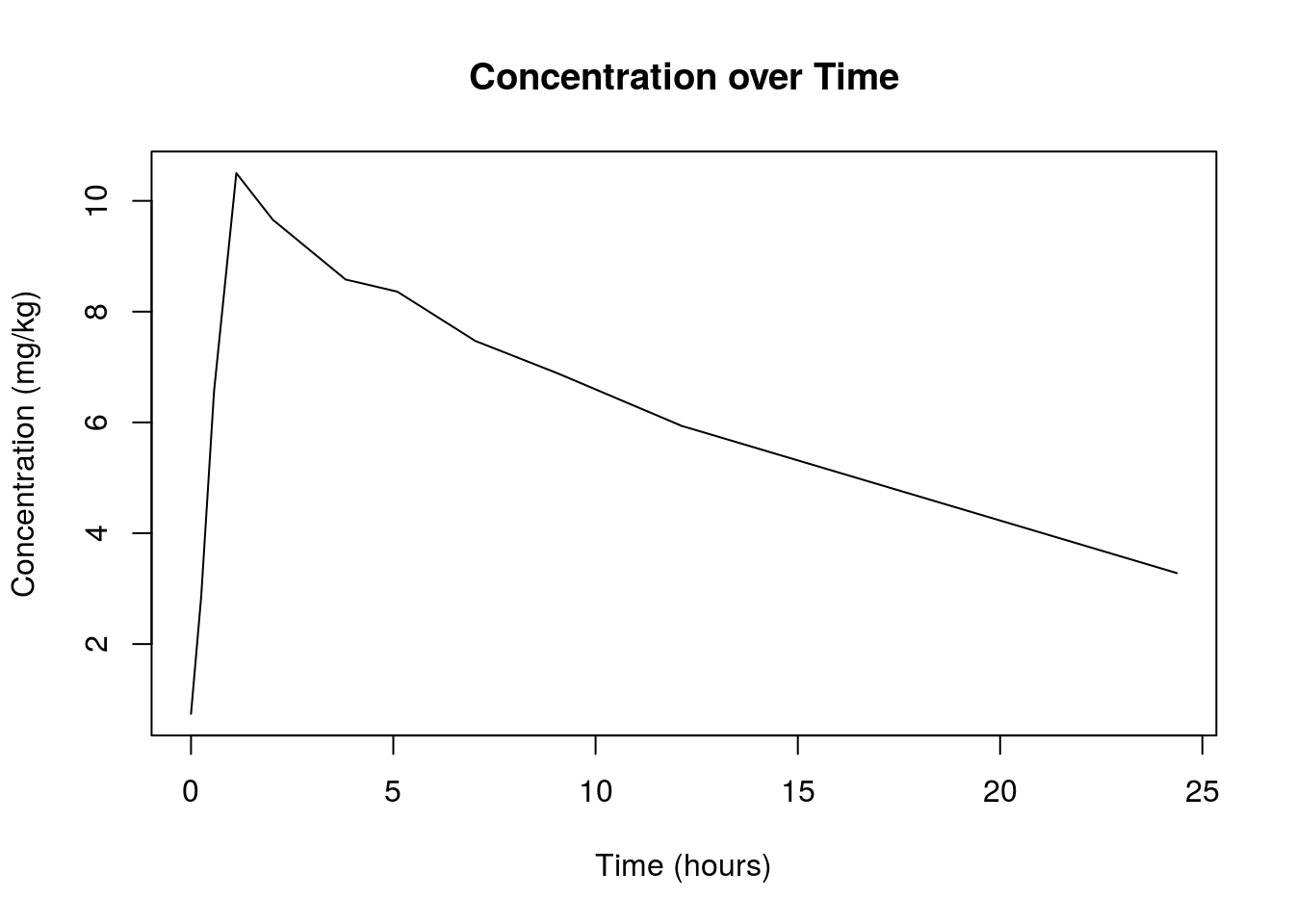Now that we’ve made our improved graph, let’s push the envelope one more time. This time we’re going to plot two sets of data on the same graph. This part gets a little tricky, so hold on to your hats! Let’s take it step by step. First, let’s plot our data just like we did before. This time, let’s also make it red using the “col” argument and setting it to “red”:

``plot(Session_1\$Time[1:11],Session_1\$conc[1:11], type="l", main="Concentration over Time", xlab="Time (hours)", ylab="Concentration (mg/kg)", col="red")``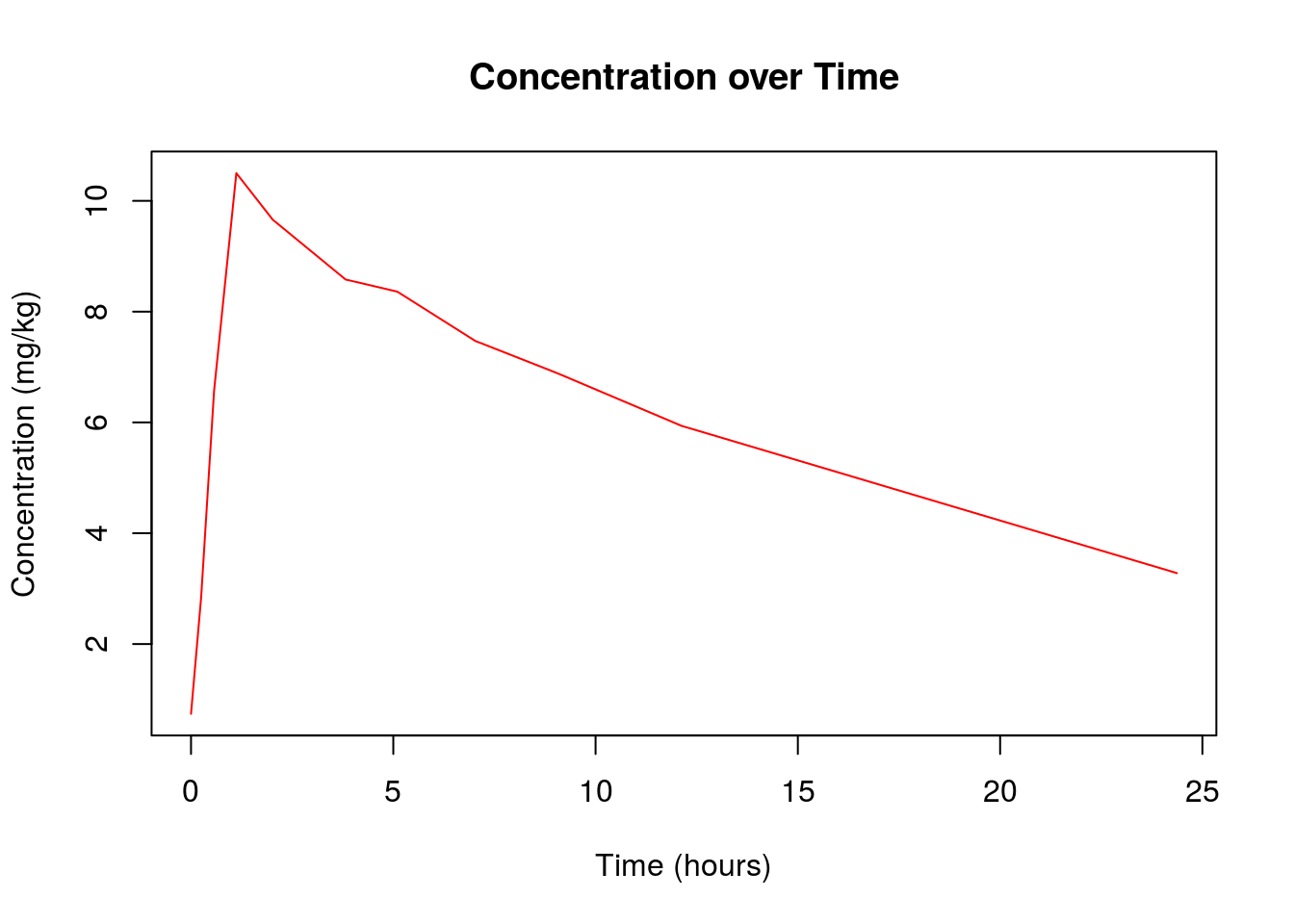Now we want to add another line plot onto this graph. For this, we will need to introduce a new function `lines()`. This function will allow you to add more data to your existing plot without erasing it! Let’s try it out with concentration data from patient 2. This time, let’s make the line blue:

``lines(Session_1\$Time[12:22],Session_1\$conc[12:22], col="blue")``

And our new and improved graph should look like this: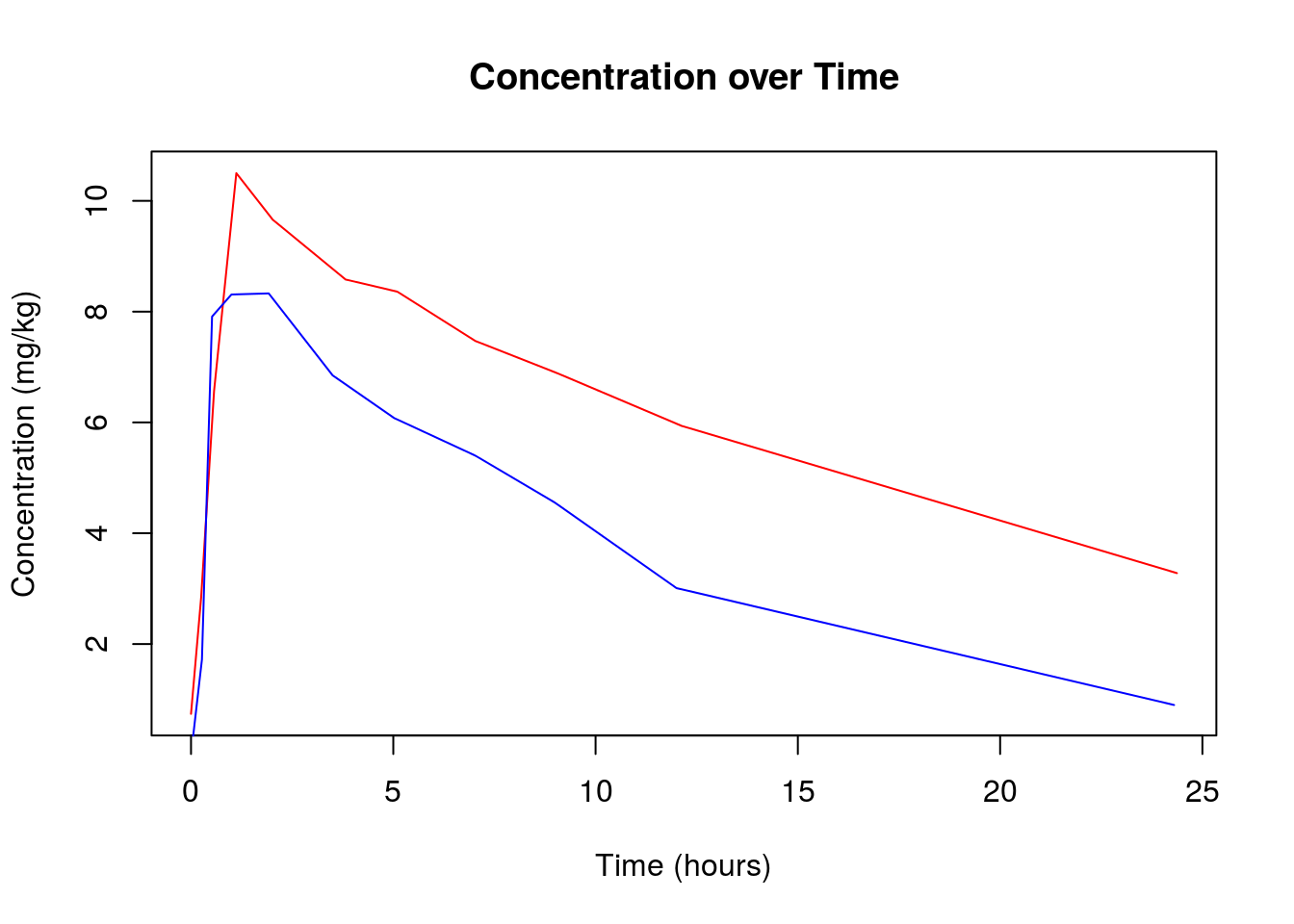Looking pretty professional! But which line corresponds to which set of data? Sounds like we could use a legend…
Let’s add one in using the `legend()` function. Here we specify an option for the position “topright”, the title “Subjects”, created a vector containing the labels “c(‘Subject 1’, ‘Subject 2’), specified the colored line widths in the legend”lwd=c(1.0,1.0)“, and colored the labels based on the order that they occur”col=c(“red”,“blue”)“.

``legend("topright",title="Subjects",c('Subject 1','Subject 2'),lwd=c(1.0,1.0),col=c("red","blue"))``

The final graph should look like this: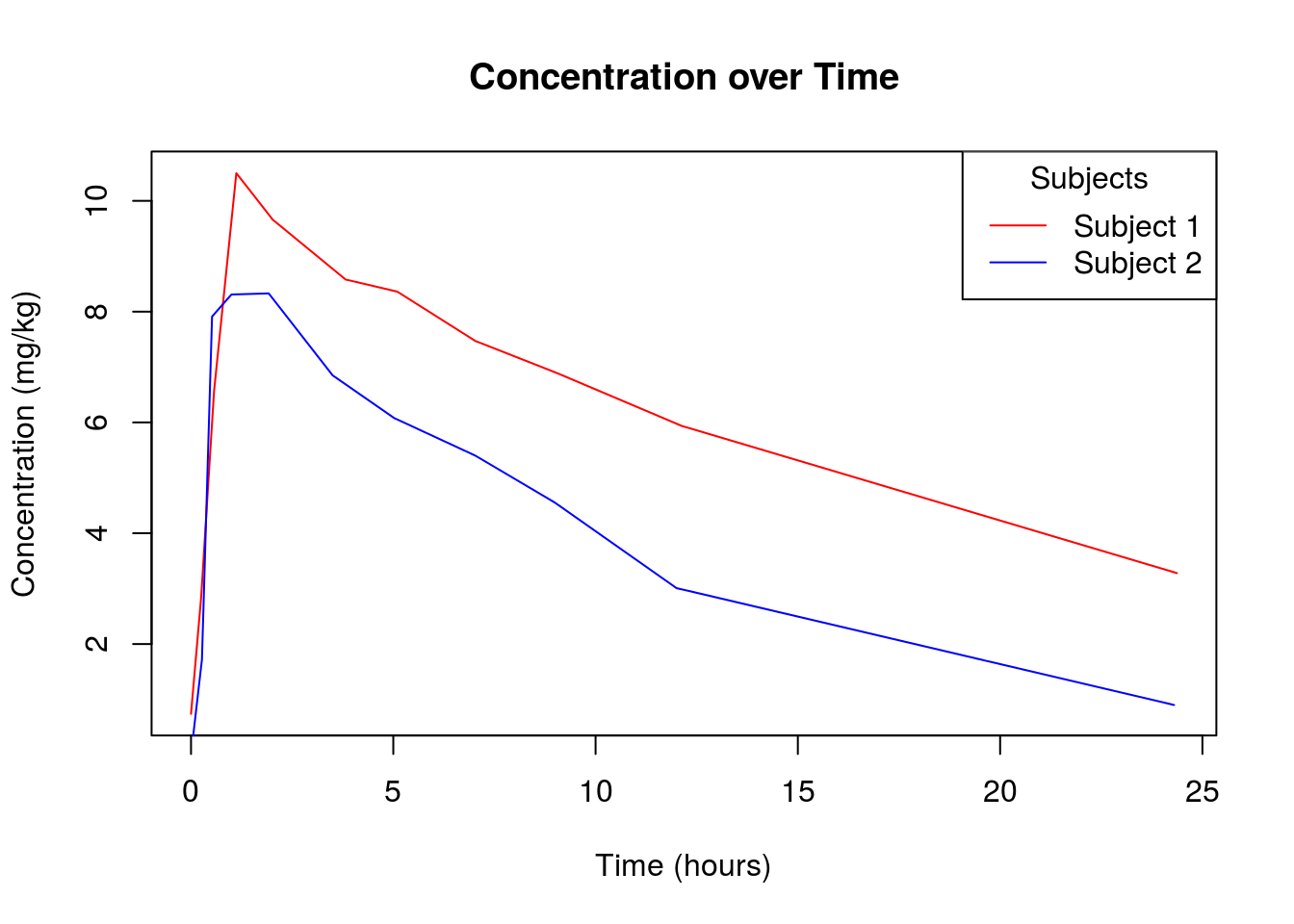You’ll learn more about plot customization in future sessions, but this should suffice for now. Let’s move on to our next type of graph, the box plot. Believe it or not, box plots are just as easy to make as line plots, they just use a different function, `boxplot()`. This time, we’re going to plot concentration data for subjects 1, 2, and 3, and make the boxes blue, green, and red, respectively. We’ve also added labels using the “names” argument.

``boxplot(Session_1\$conc[1:11],Session_1\$conc[12:22],Session_1\$conc[23:33],col=c("blue","green","red"),names=c("Subject 1","Subject 2","Subject 3"))  ``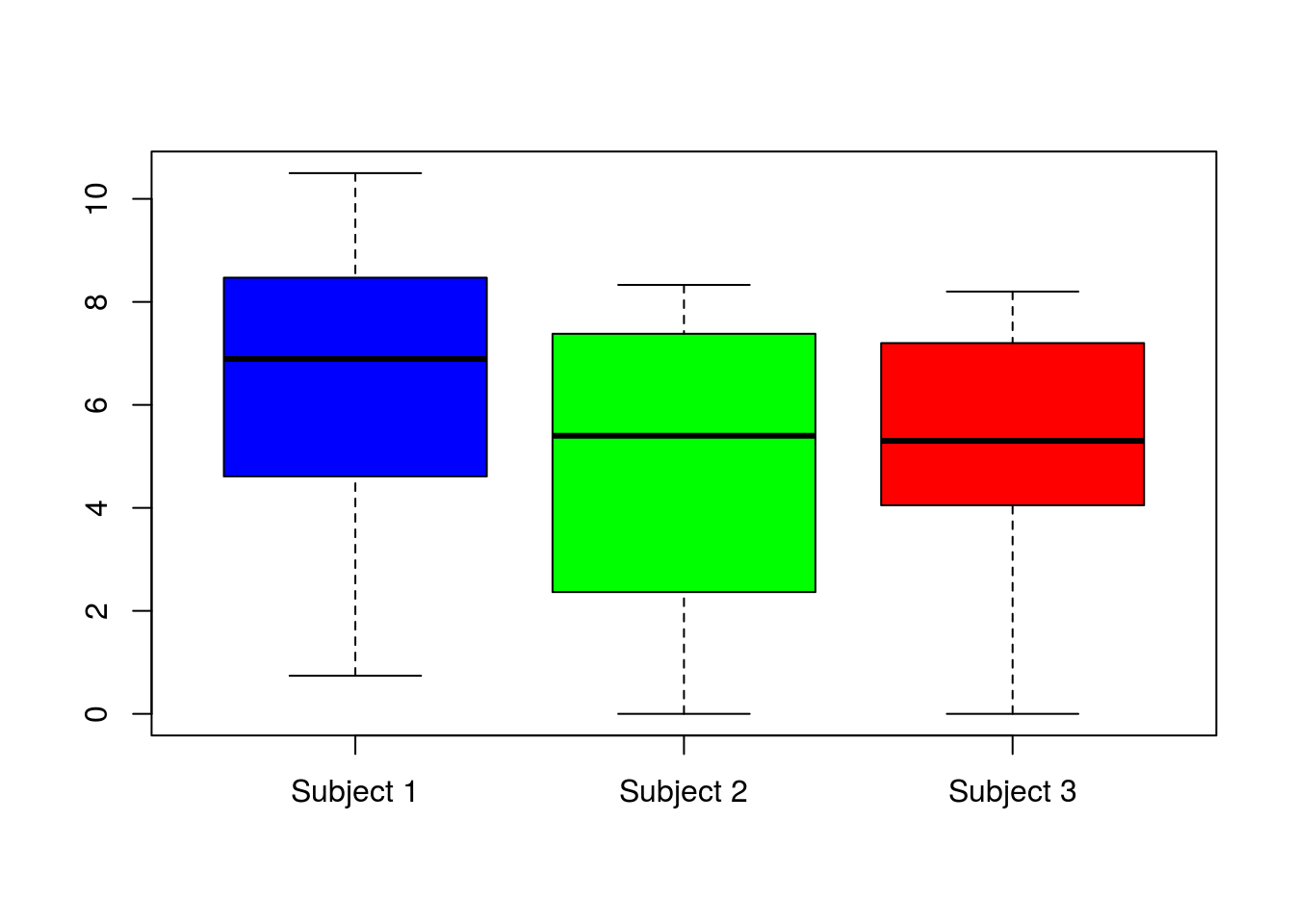The next graph we’ll be creating is the bar plot using the function `barplot()`. In this example, we’ll be plotting the maximum concentrations for the first 5 subjects over the course of the experiment. We’ll be using the `max()` function to determine the maximum value for each patient’s concentration range. The `barplot()` function will take a vector that contains our data:

``c(max(Session_1\$conc[1:11]),max(Session_1\$conc[12:22]),max(Session_1\$conc[23:33]),max(Session_1\$conc[34:44]),max(Session_1\$conc[45:55]))``

As well as a vectors that contains our ordered labels using the names argument: names=c(“Subject 1”,“Subject 2”,“Subject 3”,“Subject 4”,“Subject 5”)"
The code should look like this (we also threw in a title and a y-label):

``barplot(c(max(Session_1\$conc[1:11]),max(Session_1\$conc[12:22]),max(Session_1\$conc[23:33]),max(Session_1\$conc[34:44]),max(Session_1\$conc[45:55])), main="Maximum Concentration", names=c("Subject 1","Subject 2","Subject 3","Subject 4","Subject 5"),ylab="concentration (mg/kg)")  ``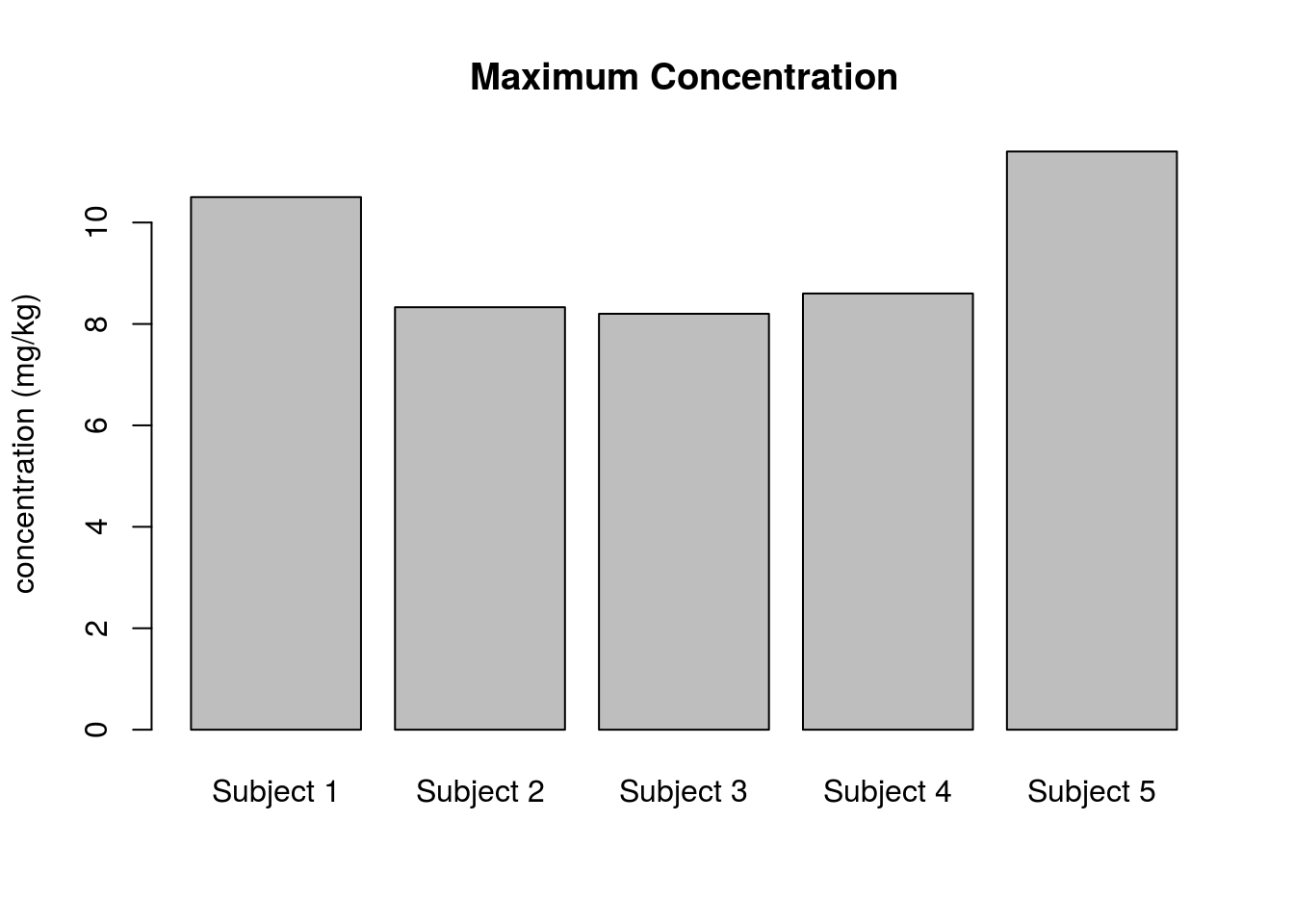Ok, those last few commands are a bit long. To make them easier to read, we can 1) store the values to be plotted in objects and 2) split them across a few lines:

``````maximums <- c(max(Session_1\$conc[1:11]),
max(Session_1\$conc[12:22]),
max(Session_1\$conc[23:33]),
max(Session_1\$conc[34:44]),
max(Session_1\$conc[45:55]))

names <- c("Subject 1","Subject 2","Subject 3","Subject 4","Subject 5")

barplot(maximums,
main="Maximum Concentration",
names=names,
ylab="concentration (mg/kg)")``````Awesome! Now let’s move on to our last type of plot, the histogram. For this data, we’ll just look at the distribution of concentrations over the experiment for all our subjects. We can do this by using the function `hist()`.
It’s easy, the code should look like this:

``hist(Session_1\$conc,main="Concentrations",xlab="Concentration Range (mg/kg)")``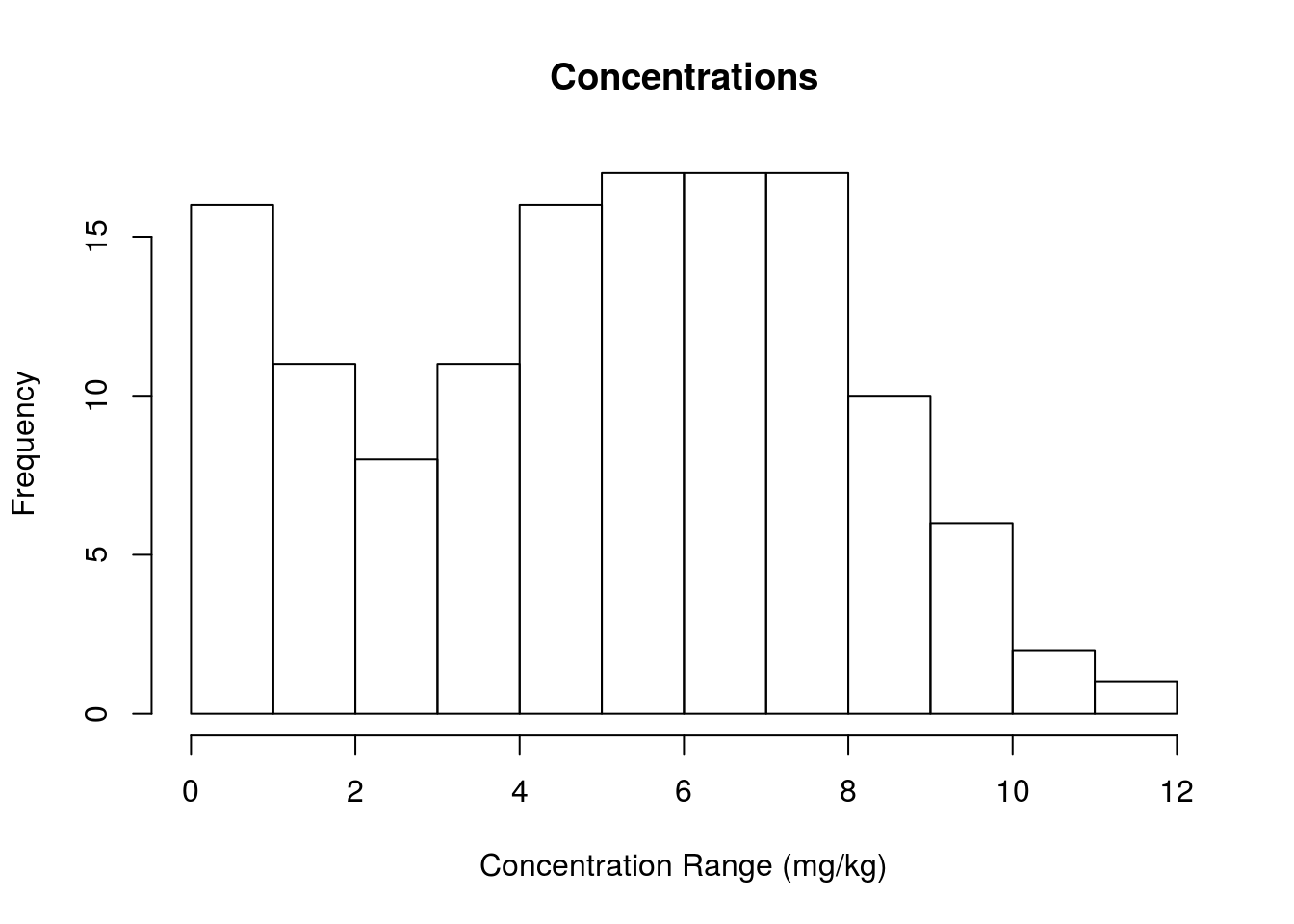That’s not bad, we have the frequency of each integer concentration up to 12. But what if we wanted a higher resolution histogram. After all, not all the concentration values are integers. We can do this by increasing the bin number using the argument breaks. Let’s modify our histogram to include 20 bins instead.

``hist(Session_1\$conc,main="Concentrations",xlab="Concentration Range (mg/kg)",breaks=20,xlim=c(0, 12))``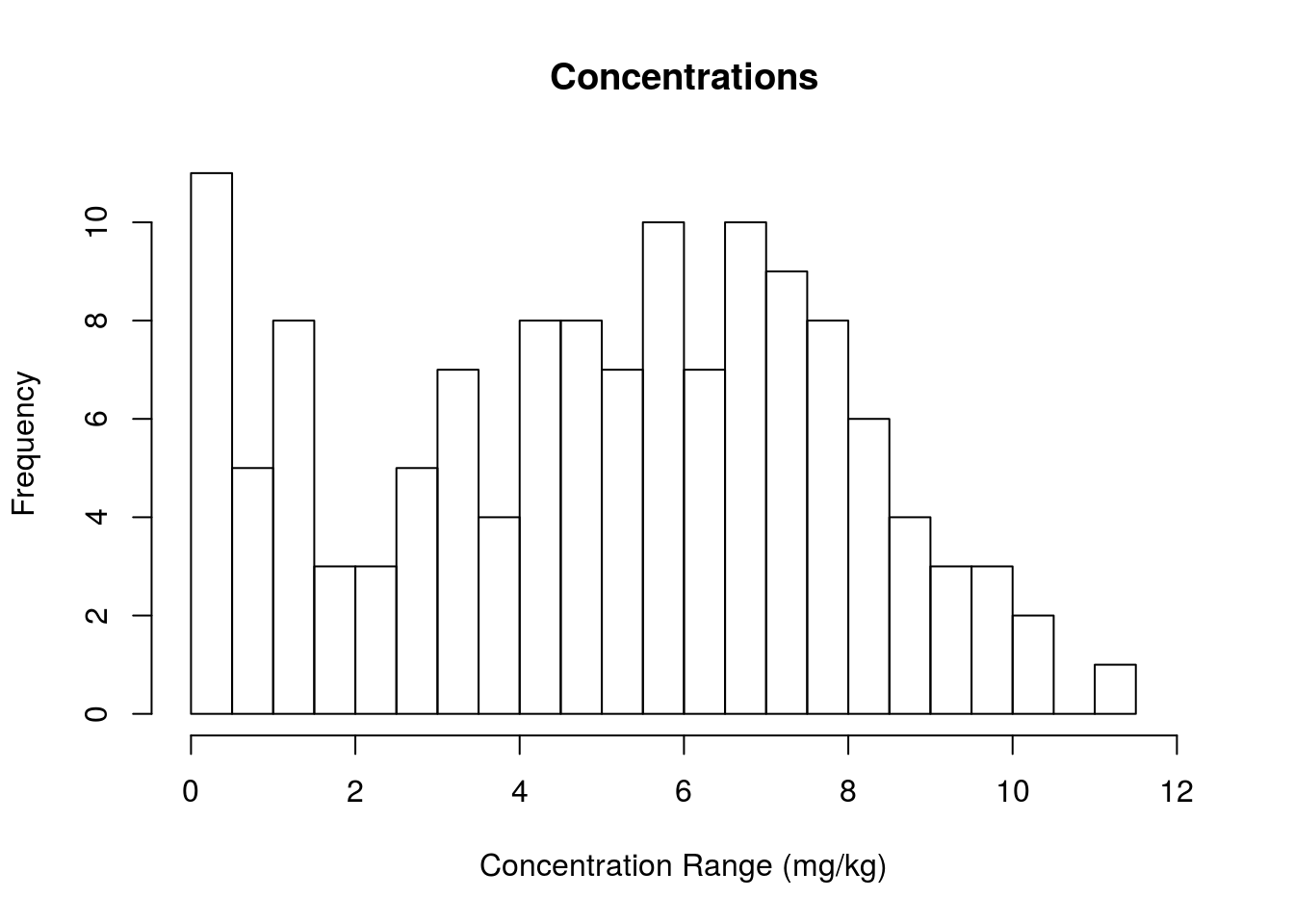Here we also changed the minimum/maximum boundaries in the x-axis to include our range of bins (without it, the x-axis would look a little wonky, see for yourself if you’re interested). We should get a histogram that looks like this:

## Summary Quiz 3

Now let’s practice your plotting skills

1. Read this file into a data frame called m: Mel.txt
2. Make a scatterplot of age versus thickness.
3. Make a box plot of thickness by status and use the following colors: darkcyan, hotpink, lawngreen (hint: use `help()` to lookup the formula method (x~y) of making box plot).
4. Plot histograms of thickness and age.
5. Plot histograms of age for men and women separately.

Advanced: Using the Session_1 data frame, make a line plot of time versus concentration for subjects 1,5,8, and 12. (hint: First use `plot()` for subject 1, then use `lines()` for the remaining. Also use `help()` to figure out how to specify the x and y axis limits using the `range()` function)

And there we have it! You did it! Welcome to the wonderful world of R plotting :-) Next session you will learn how to customize your plots to your heart’s content. For now, digest these new methods and feel free to explore different types of arguments for these functions, as there is a whole lot more to these plots if you dig a little deeper!

Use what you’ve learned about logical operators and subsetting data frames with the bracket notation (answers at end).
Back to Quiz 1
1. Is the dose for subject 1 greater than, less than, or equal to the dose for subject 2?

``Session_1[1,3] > Session_1[12,3]``
`` FALSE``
``Session_1[1,3] == Session_1[12,3]``
`` FALSE``
``Session_1[1,3] < Session_1[12,3]``
`` TRUE``
1. Dose subject 4 weigh more than subject 6?
``Session_1[34,2] > Session_1[56,2]``
`` FALSE``
``Session_1[34,2] == Session_1[56,2]``
`` FALSE``
``Session_1[34,2] < Session_1[56,2]``
`` TRUE``
1. Is the mean concentration for subject 5 greater than, less than, or equal to the mean concentration for subject 7?
``mean(Session_1[45:44,5]) > mean(Session_1[67:77,2])``
`` FALSE``
``mean(Session_1[45:44,5]) == mean(Session_1[67:77,2])``
`` FALSE``
``mean(Session_1[45:44,5]) < mean(Session_1[67:77,2])``
`` TRUE``
1. Is 4 not equal to 5 (prove it to yourself)?
``4 != 5``
`` TRUE``
1. What is the average concentration for Subject 7?
``mean(Session_1[Session_1\$Subject == 7, "conc"])``
`` 3.910909``
1. What is the minimum concentration when the time is greater than 5 minutes?
``minConc <- min(Session_1[Session_1\$Time > 5, "conc"])``
1. Which subject corresponds to the minimum concentration found in #6?
``Session_1[Session_1\$conc == minConc, "Subject"]``
`` 11``

Back to Quiz 1

Here are a few more questions to practice sorting data
Back to Quiz 2
1. Sort the Session_1 data frame by the Time variable and store it in a new object called sorted.

``sorted <- Session_1[order(Session_1\$Time),]``
1. Sort the Session_1 data frame by the Dose and conc variables and store it in a new object called sorted2.
``sorted2 <- Session_1[order(Session_1\$Dose,Session_1\$conc),]``
1. Sort the Session_1 data frame from the largest to smallest values and store it in a new object called sorted3.
``sorted3 <- Session_1[order(Session_1\$conc),]``

Back to Quiz 2

Now let’s practice your plotting skills
Back to Quiz 3
1. Read this file into a data frame called m: Mel.txt

``m <- read.delim(file.choose())``
1. Make a scatterplot of age versus thickness.
``plot(m\$age,m\$thickness)``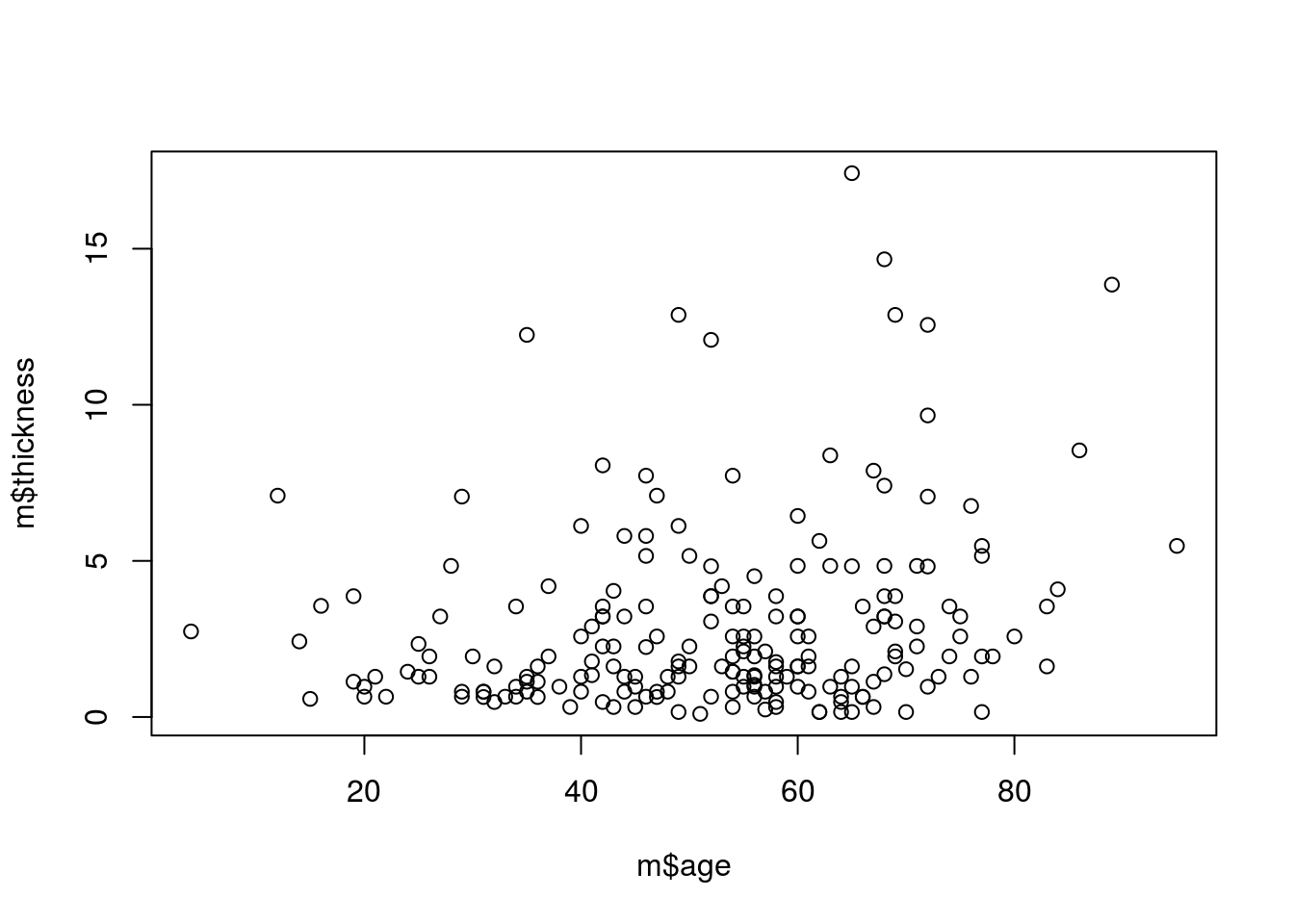1. Make a box plot of thickness by status (hint: use `help()` to lookup the formula method (x~y) of making box plot).
``````statusColors <- c("darkcyan","hotpink","lawngreen")
boxplot(m\$thickness ~ m\$status, col=statusColors)``````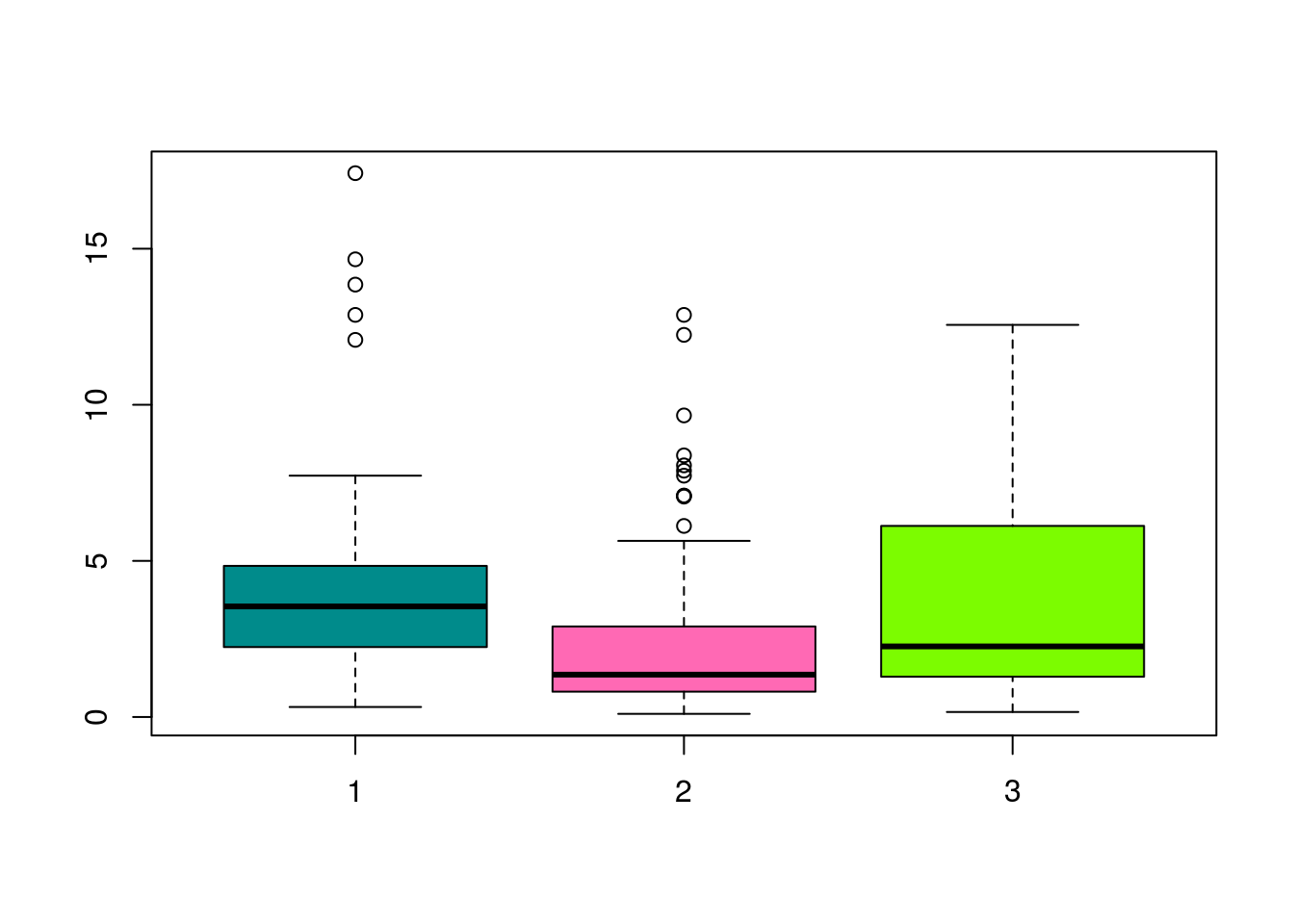1. Plot histograms of thickness and age.
``hist(m\$thickness)``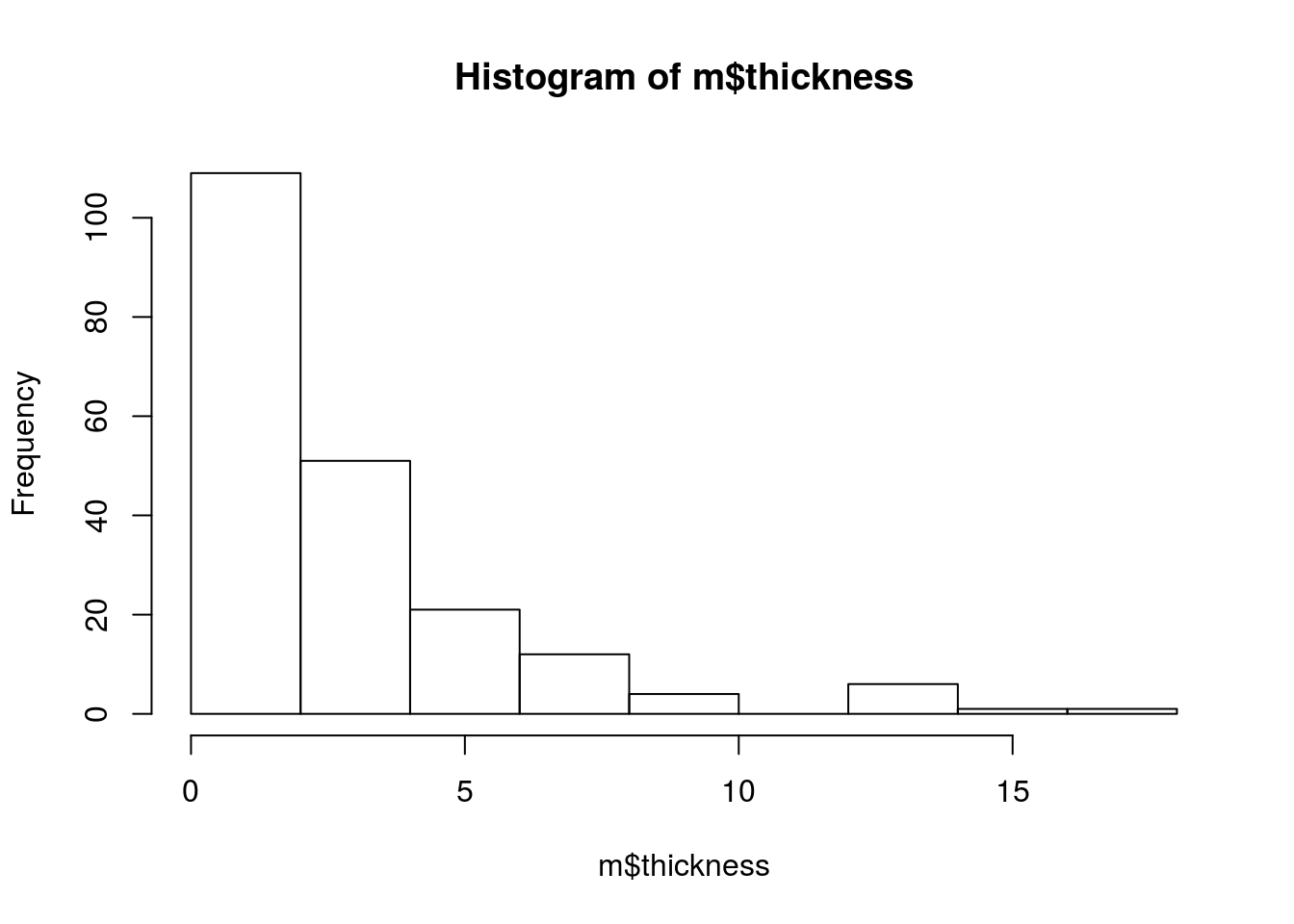``hist(m\$age)``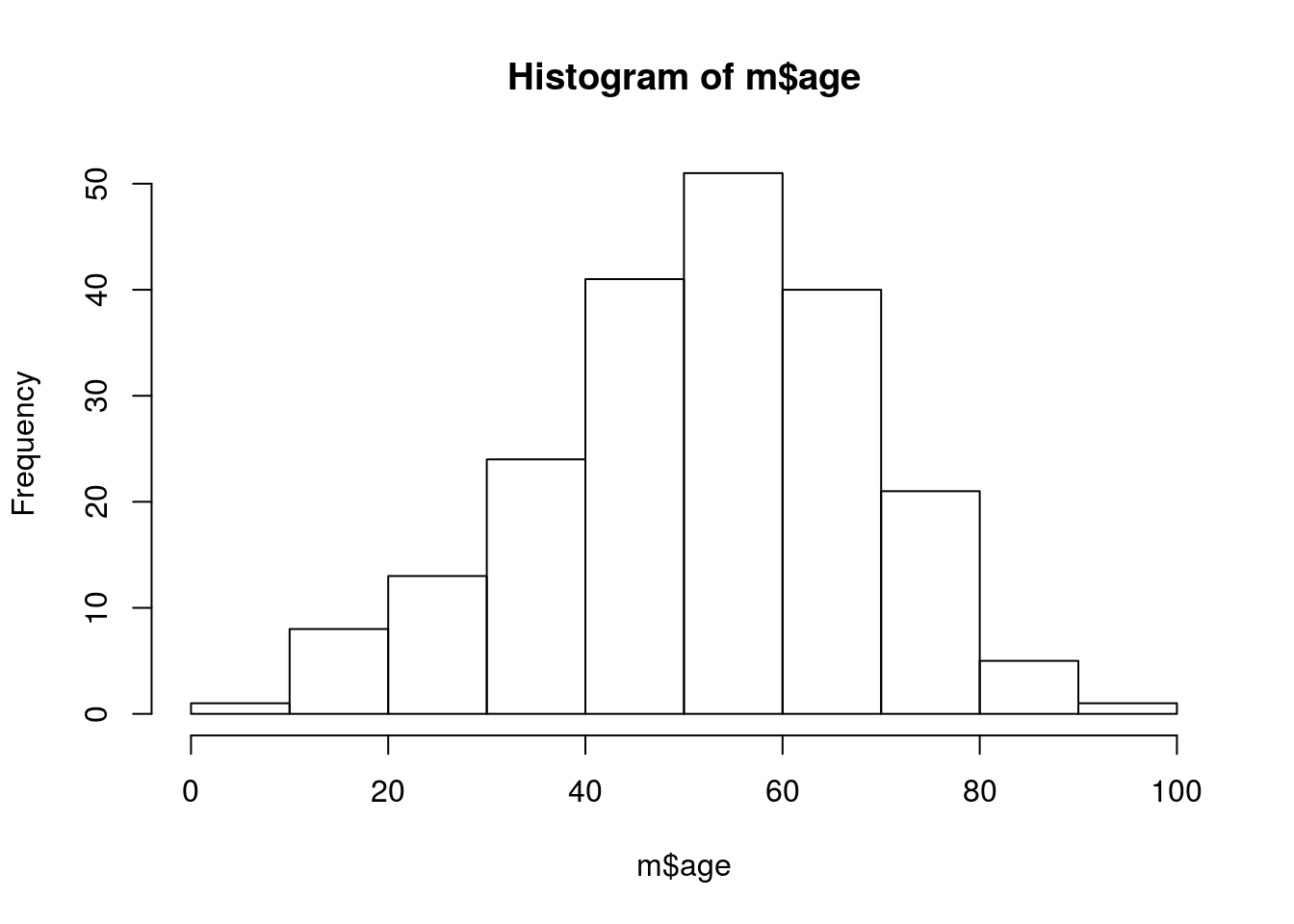1. Plot histograms of age for men and women separately (hint: m\(age[m\)sex == 1]).
``hist(m\$age[m\$sex == 1])``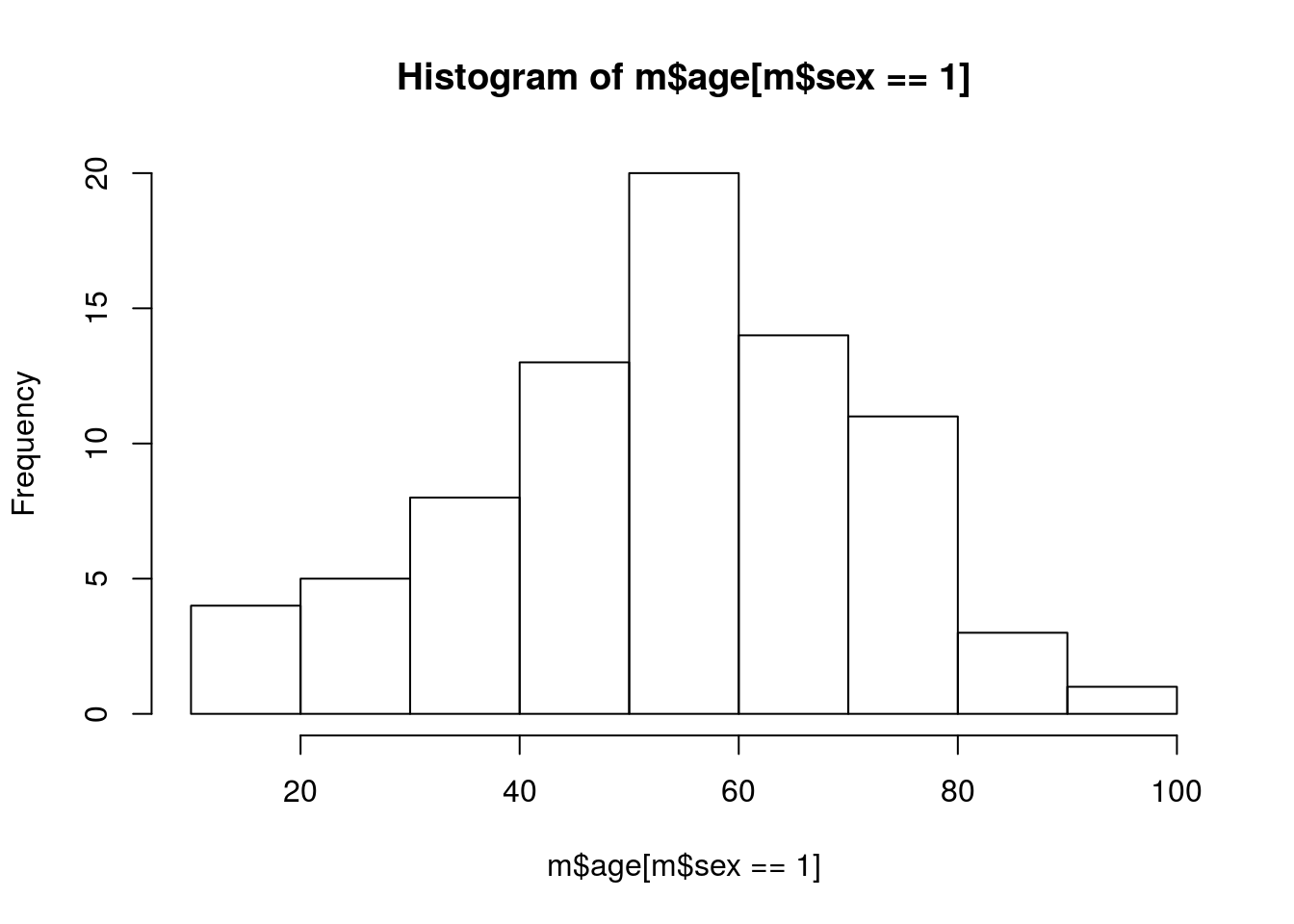``hist(m\$age[m\$sex == 0])``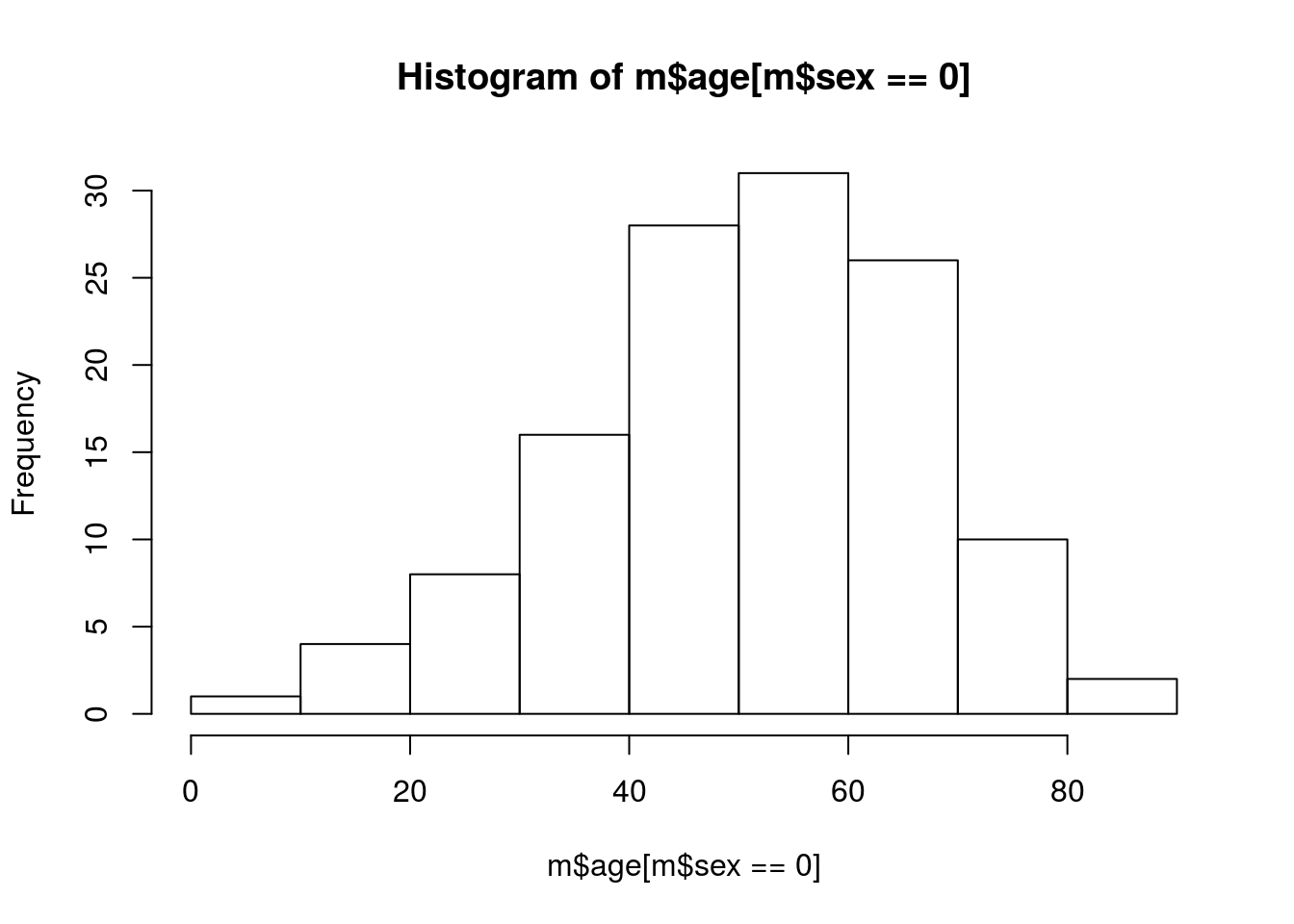``````plot(m\$age,m\$thickness,
main="Chart title",
xlab="Age",
ylab="Thickness")``````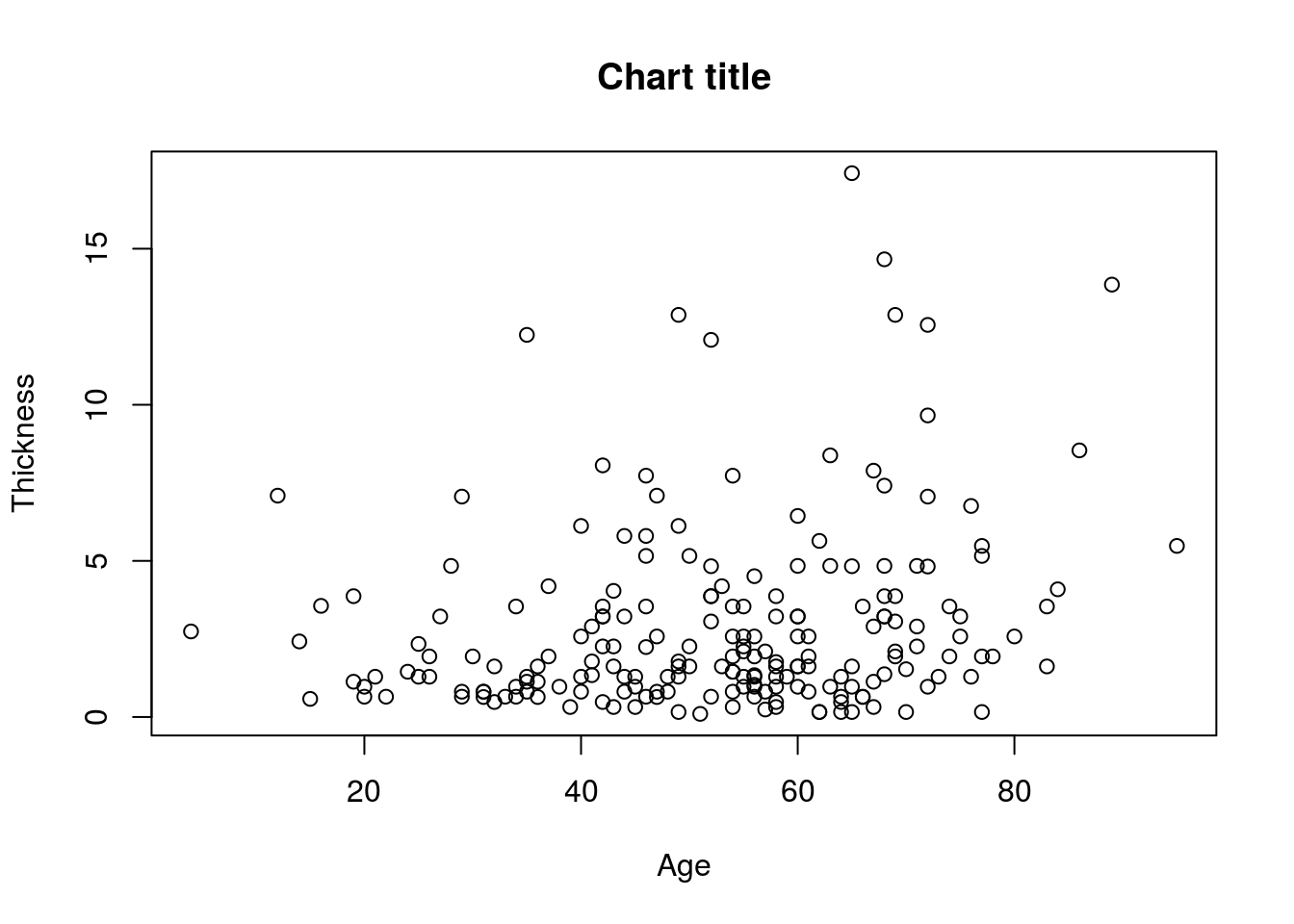Back to Quiz 3

Advanced: Using the Session_1 data frame, make a line plot of time versus concentration for subjects 1,5,8, and 12. (hint: First use `plot()` for subject 1, then use `lines()` for the remaining. Also use `help()` to figure out how to specify the x and y axis limits using the `range()` function). Note: this is an advanced solution and we’ll learn more about it in the next session but try to understand the code as best you can. In particular, understand what paste(“Subjects”, plotSubjects), plotSubjects, plotColors are doing.

``````## Make some vectors that will help us plot
plotColors    <- c("olivedrab","steelblue","darkmagenta","burlywood4")
plotSubjects  <- c(1,5,8,12)
lineNames     <- paste("Subjects", plotSubjects)

## Plot the first line for subject 1
plot(Session_1\$Time[Session_1\$Subject == plotSubjects],
Session_1\$conc[Session_1\$Subject == plotSubjects],
type="l",
col=plotColors,
xlim=range(Session_1\$Time),
ylim=range(Session_1\$conc),
main="Concentration over Time for Subjects 1, 5, 8, and 12",
xlab="Time",
ylab="Concentration")``````